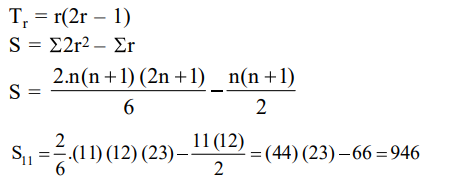# Solve this followingQuestion:

The sum of the series $1+2 \times 3+3 \times 5+4 \times 7+\ldots$

upto $11^{\text {th }}$ term is :-

1. 915

2. 946

3. 945

4. 916

Correct Option: , 2

Solution: